• JAVA 使用栈实现中缀表达式转换后缀表达式一文中详细说明了如何将中缀表达式转换成后缀表达式。 那么如何计算后缀表达式的结果呢？ 后缀表达式的求值思路： 1、从左到右扫描后缀表达式 2、如果遇到操作数，将其...
JAVA 使用栈实现中缀表达式转换后缀表达式 一文中详细说明了如何将中缀表达式转换成后缀表达式。

那么如何计算后缀表达式的结果呢？

后缀表达式的求值思路：

1、从左到右扫描后缀表达式

2、如果遇到操作数，将其压入栈中。

3、如果遇到操作符，则从栈中弹出两个操作数，计算结果，然后把结果入栈。

4、遍历完后缀表达式，则计算完成，此时的栈顶元素即为计算结果。

例如：后缀表达式"3 4 + 5 × 6 - "

(1) 初始化栈，栈顶指针为空，从左至右扫描；

(2) 遇到操作数3，入栈；

(3) 遇到操作数4，入栈；

(4) 遇到操作符+，弹出栈中两个元素4，3（4 为栈顶元素，3 为次顶元素），计算结果7入栈;

(5) 遇到操作数5，入栈；

(6) 遇到运算符*，弹出栈中两个元素5，7，并计算5*7=35，计算结果35入栈 ；

(7) 遇到操作数6，入栈；

(8)  遇到操作符-，弹出栈中两个元素35，6，并计算35-6=29，计算结果入栈29，由此得出最终结果。

本文，需要先定义一个后缀表达式，为了方便数字和符号使用空格隔开。

代码思路：

1. 先将 "3 4 + 5 × 6 - "  放入到List 中 。

//将字符串转换成List
public static List<String> stringToList(String suffixExpression){
List<String> list = new ArrayList<String>();
String[] sts = suffixExpression.split(" ");
for(String s:sts){
list.add(s);
}
return list;
}

2. 将 ArrayList 传递给cal方法，遍历 ArrayList 配合栈 完成计算。

public  static int cal(List<String> list){
Stack<String> stack = new Stack<String>();
int res = 0;
for(String item:list){
if(item.matches("\\d+")){
stack.push(item);
}else{
int num1 = Integer.parseInt(stack.pop());
int num2= Integer.parseInt(stack.pop());
if(item.equals("+")){
res = num2+num1;
}else if(item.equals("-")){
res = num2-num1;
}else if(item.equals("*")){
res = num2*num1;
}else if(item.equals("/")){
res = num2/num1;
}else {
throw new RuntimeException("运算符有误~~~~~~~~~~~~~~");
}
stack.push(res+"");
}
}
return Integer.parseInt(stack.pop());
}

输出结果：

后缀表达式对应的List=[3, 4, +, 5, *, 6, -]
计算的结果是=29

展开全文• 后缀表达式表示为：8 4 + 6 2 * - 例题2 中缀表达式：（70 + 30） *20 + 10 / 2 - 3 后缀表达式： 70 30 + 20 * 10 2 / + 3 - 例题3 中缀表达式： 10 * 3 +（3-1）* 2 - 10 / 2 后缀表达式：10 3 * 3 1 - 2 * + 10 2...
例子
例题1
中缀表达式“8+4-6*2”
后缀表达式表示为：8 4 + 6 2 * -
例题2
中缀表达式：（70 + 30） *20 + 10 / 2 - 3
后缀表达式： 70 30 + 20 * 10 2 / + 3 -
例题3
中缀表达式： 10 * 3 +（3-1）* 2 - 10 / 2
后缀表达式：10 3 * 3 1 - 2 * + 10 2 / -
例题3：
中缀表达式“2*(3+5)+7/1-4
后缀表达式表示为：3 5 + 2 * 7 1 /4 - +
注意：当前没有小数点，只有加减乘除
题目解析
初始化一个栈。
从左到右扫描后缀表达式中的符号，有两种情况：
如果是数字，则直接压栈。
如果是运算符op，则从栈顶弹出两个元素a和b（b为栈顶元素），然后将(a op b)压栈。
    public static String back_medium(String[] strs){
Stack<String> stack = new Stack<>();
for (int i = 0; i < strs.length; i++){
if (Character.isDigit(strs[i].charAt(0))){ // 是数字
stack.push(strs[i]);
}else{  // 不是数字
if (stack.size() < 2){
throw new RuntimeException("表达式错误");
}
// 从栈底到栈顶
String top = stack.pop();
String button = stack.pop();
String t = "(" + button+ strs[i] + top + ")";
stack.push(t);
}
}

if (stack.empty()){
throw new RuntimeException("表达式错误");
}

return stack.pop();
}

public static void main(String[] args) {
System.out.println(back_medium(new String[]{"70", "30", "+", "20", "*", "10", "2", "/", "+", "3", "-"}));
}



展开全文• // 栈实现后缀表达式求值 /* 算法思想： 遍历整个表达式 如果是操作数，入栈； 如果是操作符，将当前栈顶元素和栈第二个元素出栈进行运算，并将结果压栈；若是除（减）操作符，第二个元素作为被除数（被减数），栈顶...


算法思想：

遍历整个表达式
如果是操作数，入栈；
如果是操作符，将当前栈顶元素和栈第二个元素出栈进行运算，并将结果压栈；若是除（减）操作符，第二个元素作为被除数（被减数），栈顶元素作为除数（减数）；
表达式遍历完后，当前栈的栈顶元素即为所求表达式的值。

代码如下：

// ps:本示例代码只对10以内的整数有效
#include "stdafx.h"
#include<iostream>
using namespace std;
double val(double a, char op, double b)
{
switch (op)
{
case '+': return a + b;
case '-':return a - b;
case '*':return a*b;
case'/':
if (b == 0)
{
cout << "ERROR" << endl;
return 0;
}
else
return a / b;
default:
cout << "ERROR" << endl;
return 0;
}
}
int _tmain(int argc, _TCHAR* argv[])
{
double Stack;
int top = -1;

char tt[] = { '4','5', '*', '9','+','3','4','2','/','8','6','*','-','+','/' };
for (int i = 0; i < sizeof(tt) / sizeof(char); ++i)
{
if (tt[i] != '+' && tt[i] != '-' && tt[i] != '*' && tt[i] != '/')
Stack[++top] = tt[i]- '0';//如果是操作数，入栈, tt[i]-'0'将字符转换为整型(只对10以内的整数有效)
else
{
int b = Stack[top--];//取出当前栈顶元素
int a = Stack[top--];//取出当前栈第二个元素
Stack[++top] = val(a, tt[i], b);//进行运算，并将运算结果压栈
//cout << a << tt[i]<< b << '=' << Stack[top] << endl;
}
}

cout << Stack[top--]<<endl;//打印最后结果

return 0;
}



展开全文算法
• 先看下面的图片，这是后缀表达式32/6*3-3+转前缀的过程 对于表达式：32/6*3-3+ 其前缀式为：+-*/32633 操作数的顺序不会改变，变的是运算符的顺序，并且在后缀式中，运算顺序从左往...
先看下面的图片，这是后缀表达式32/6*3-3+转前缀的过程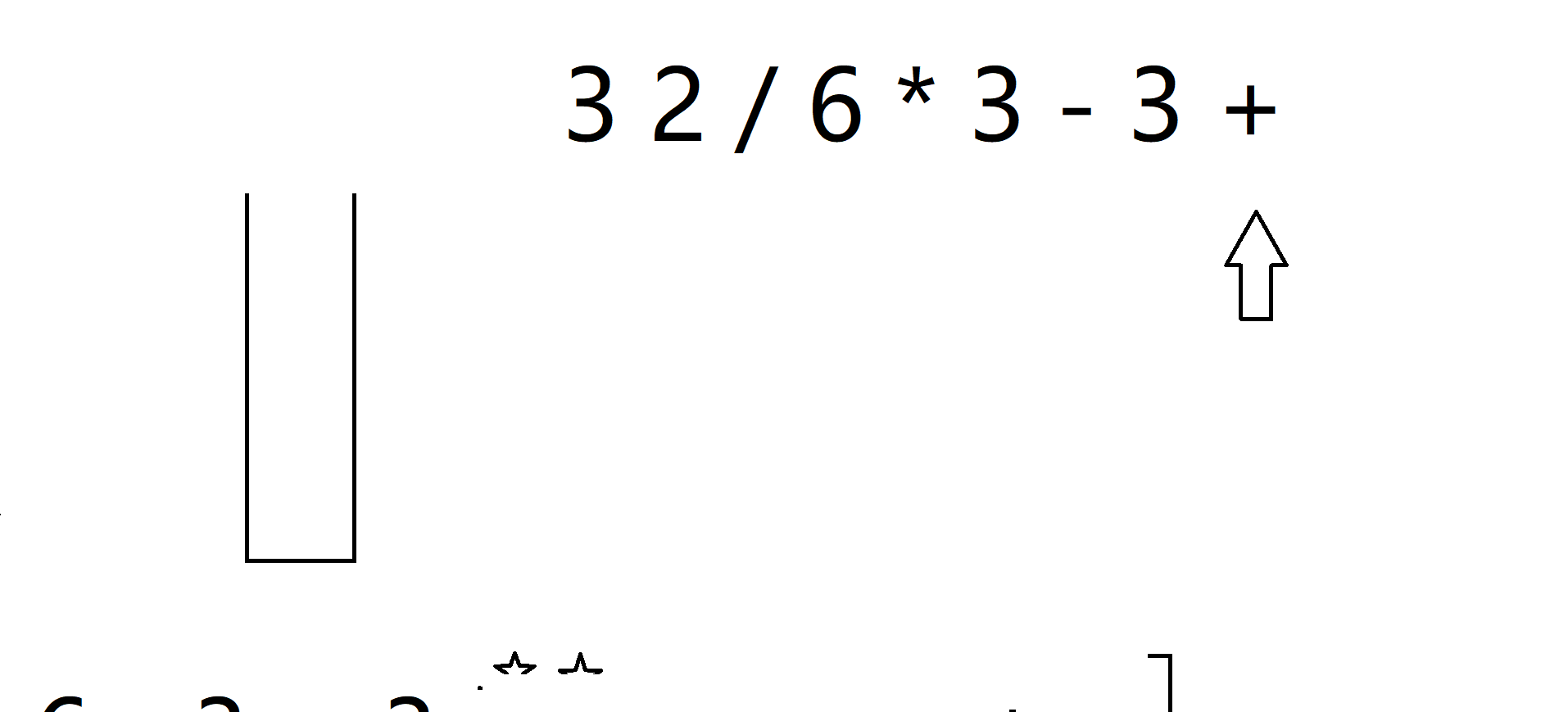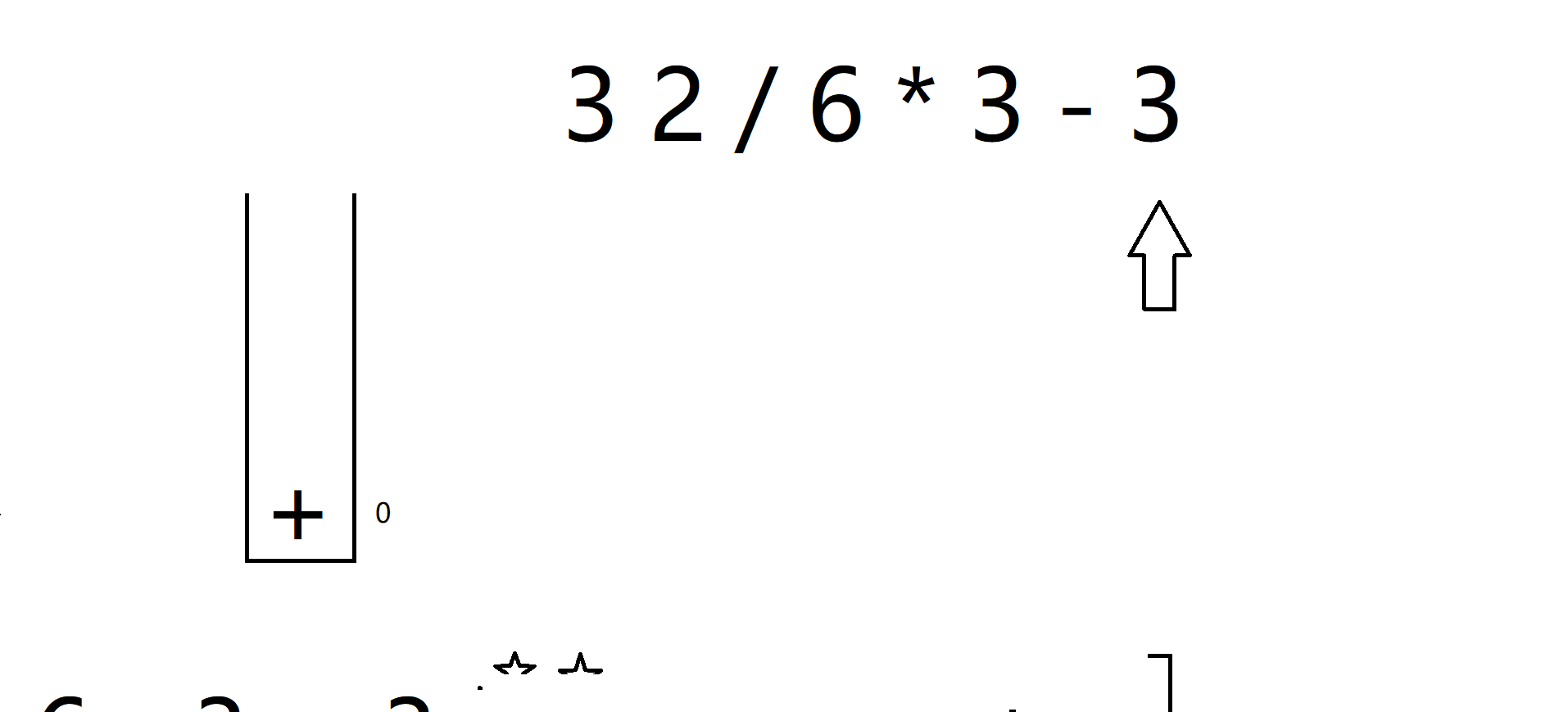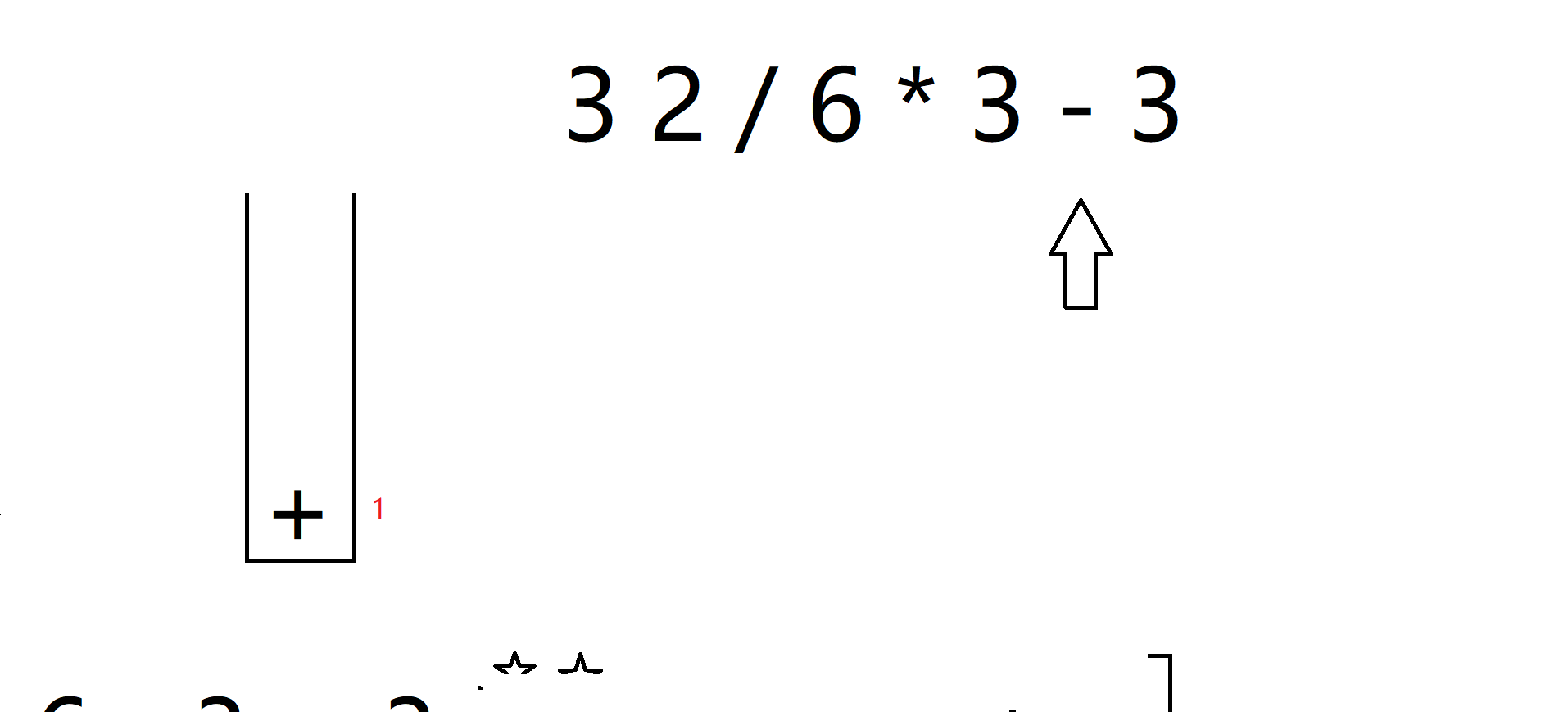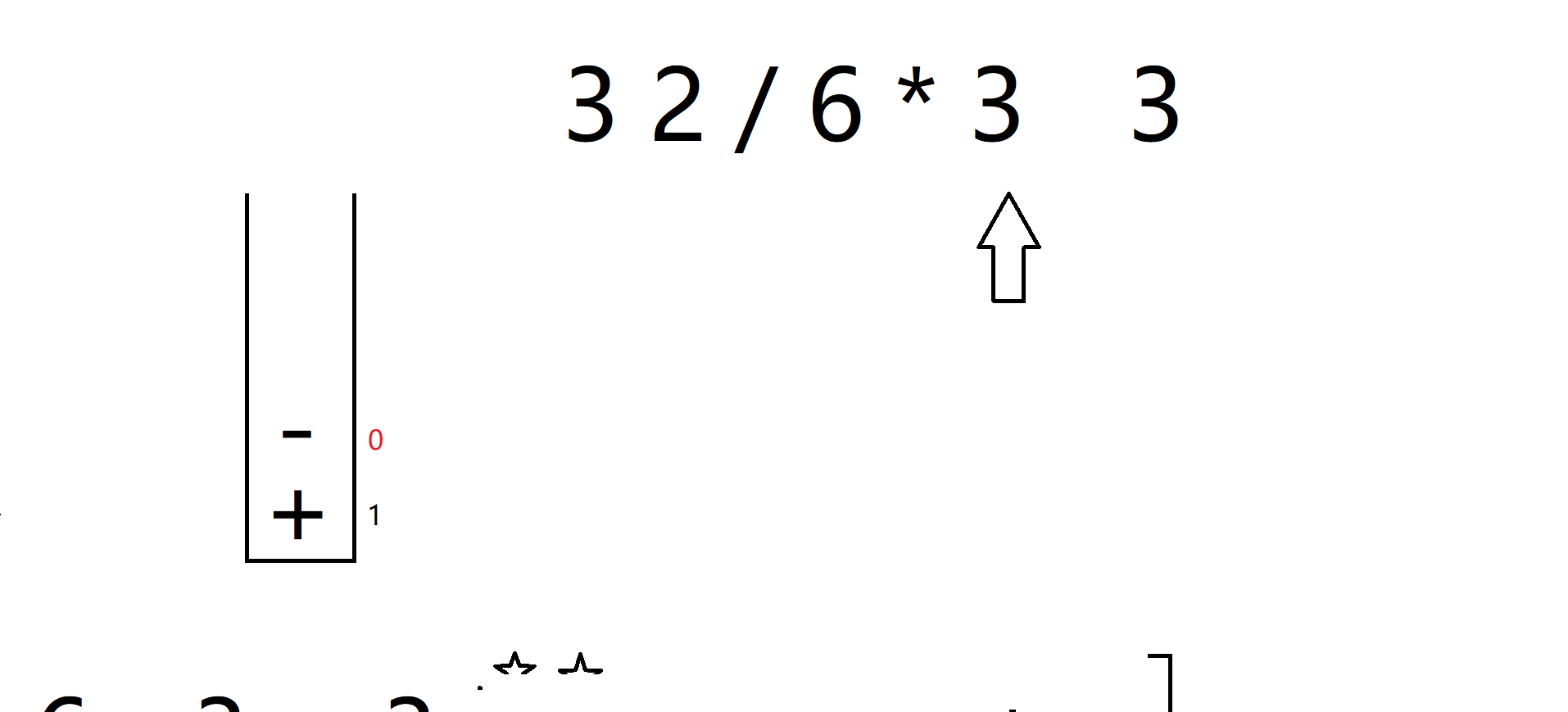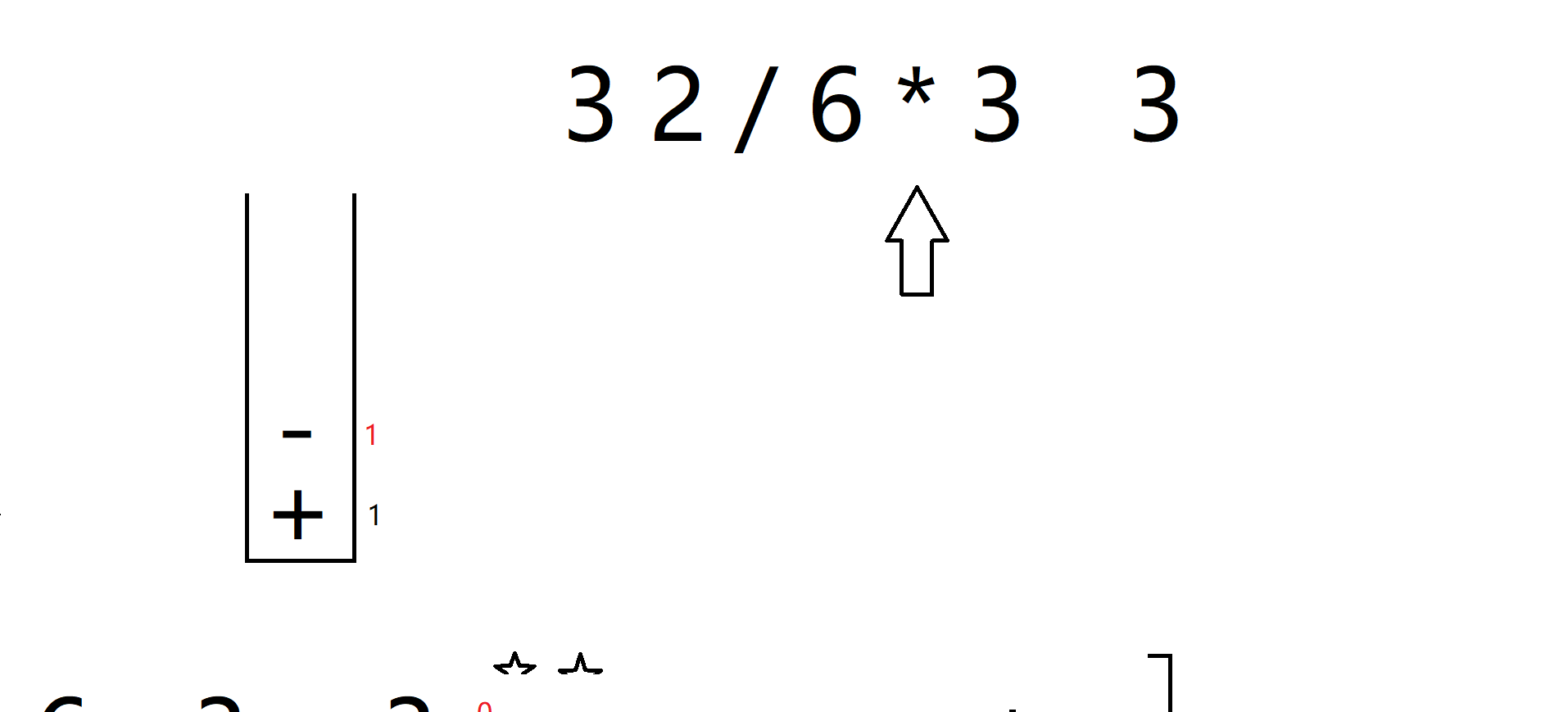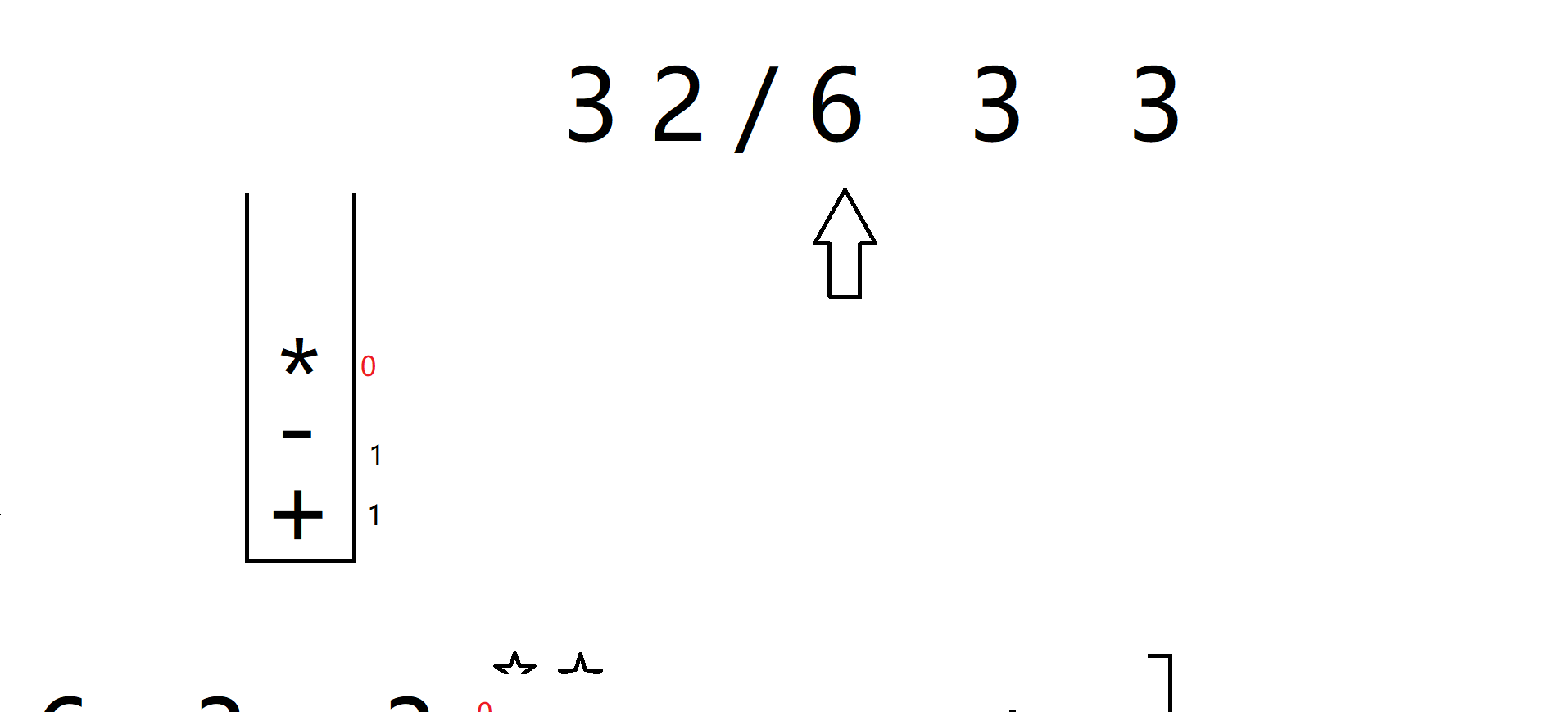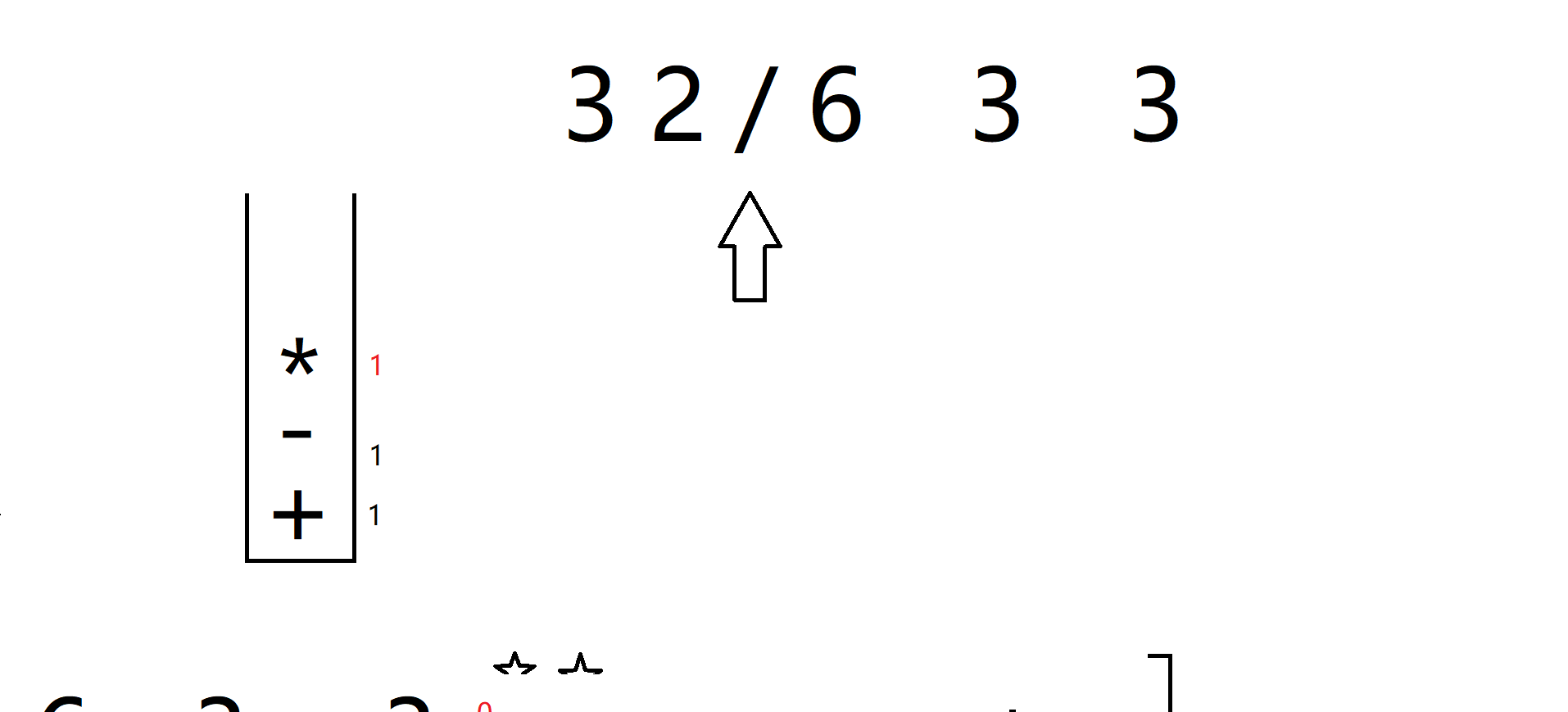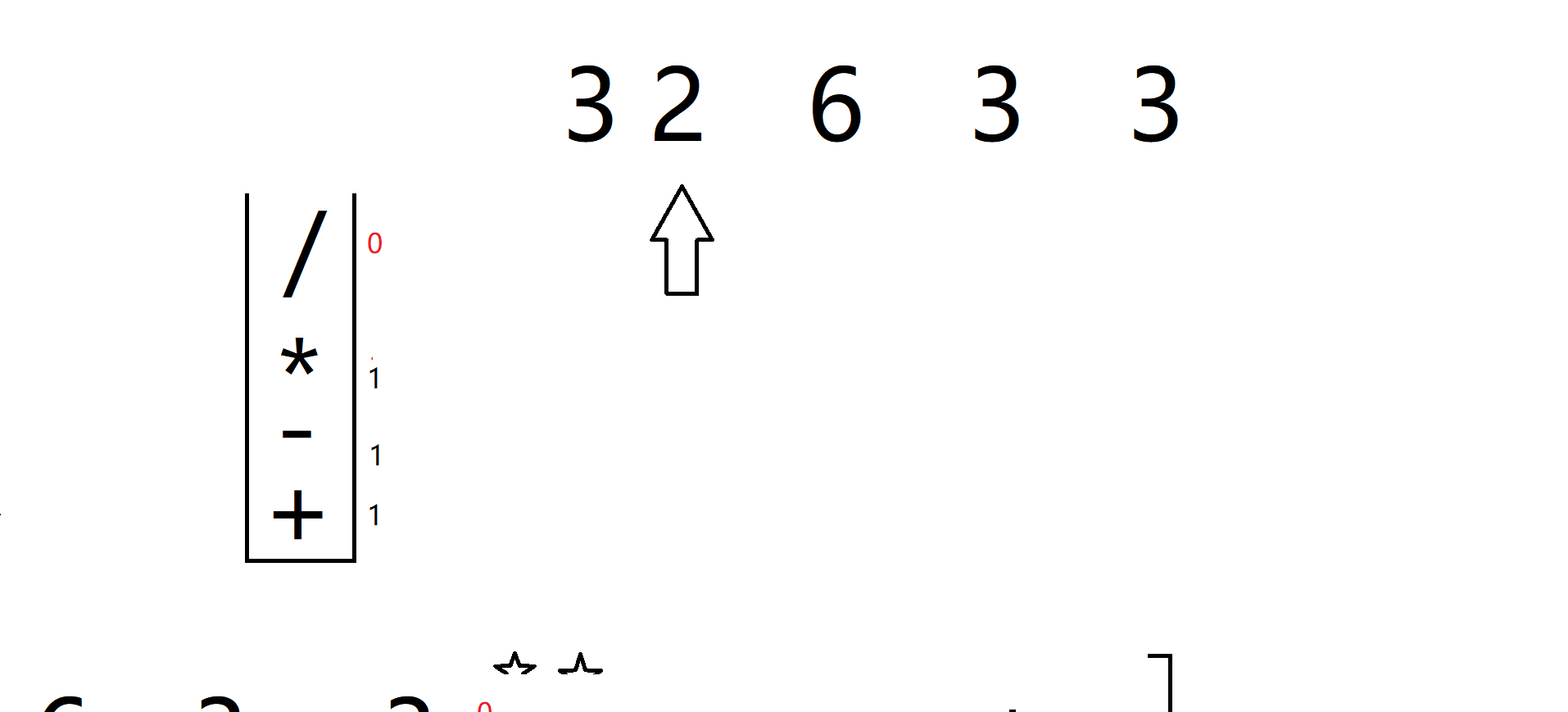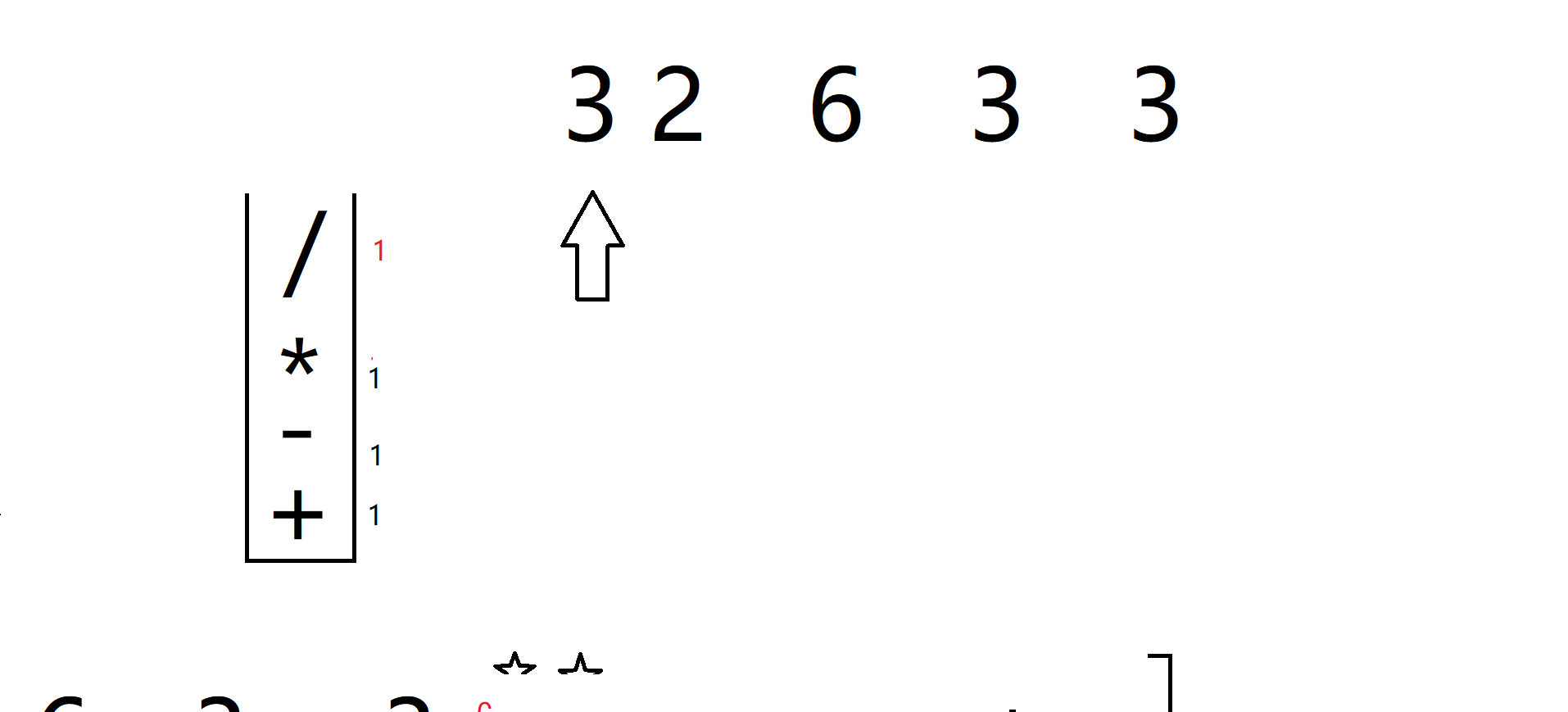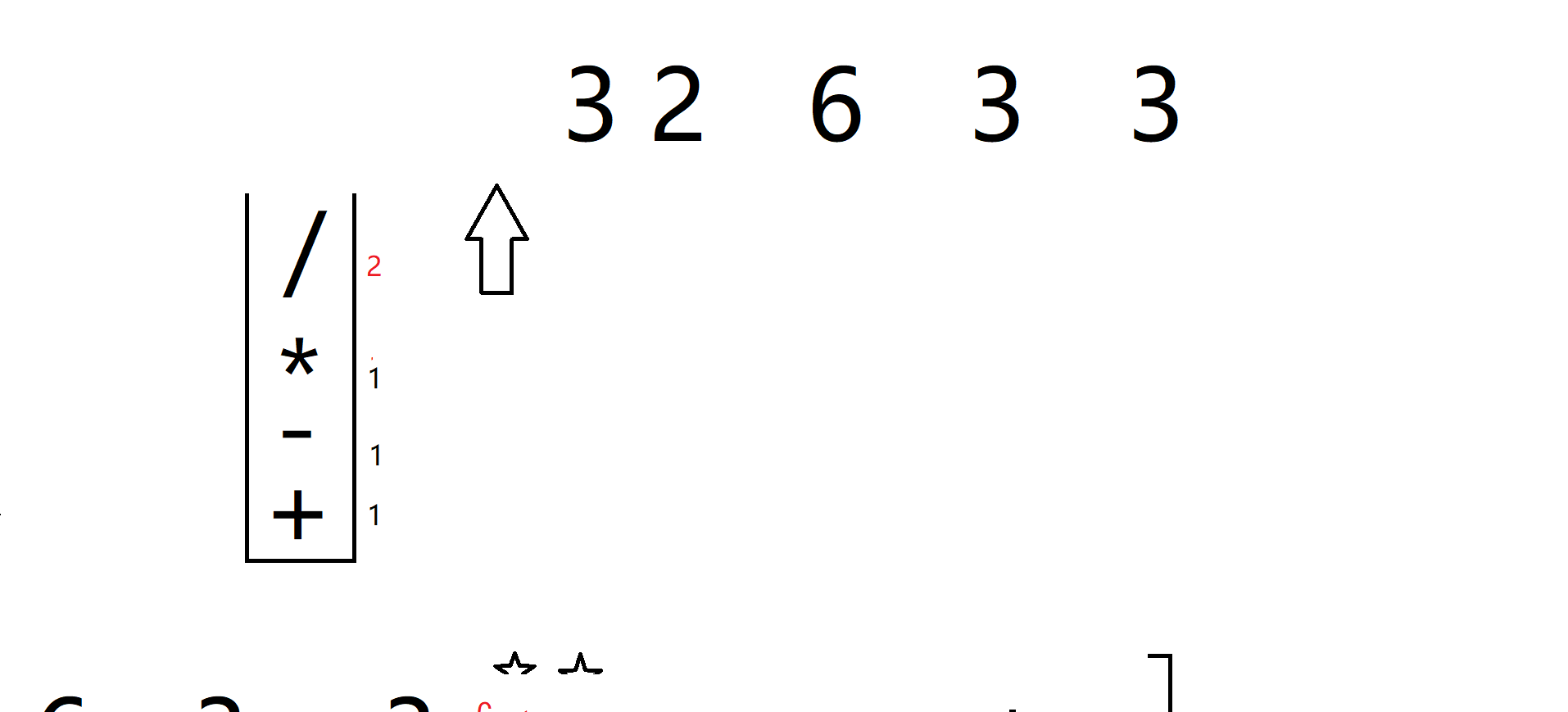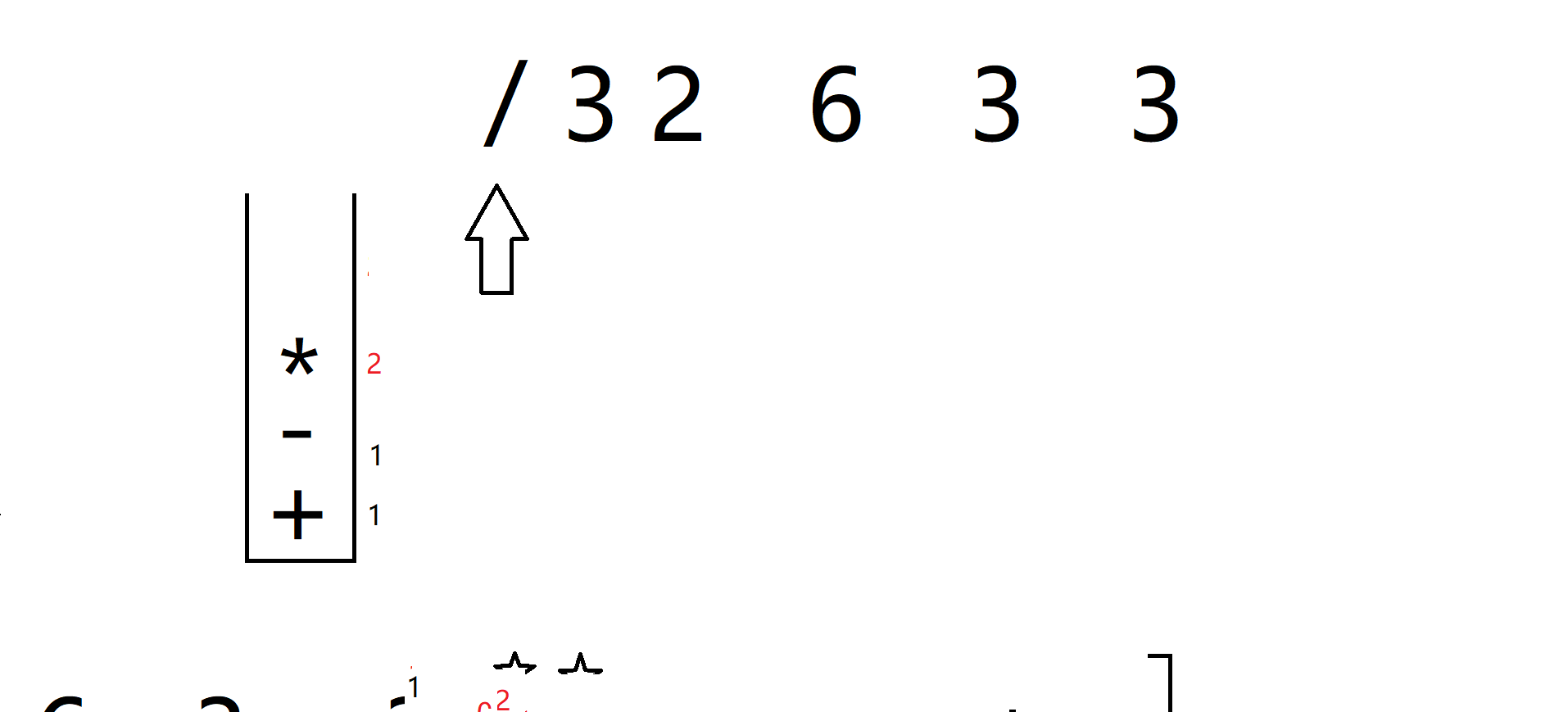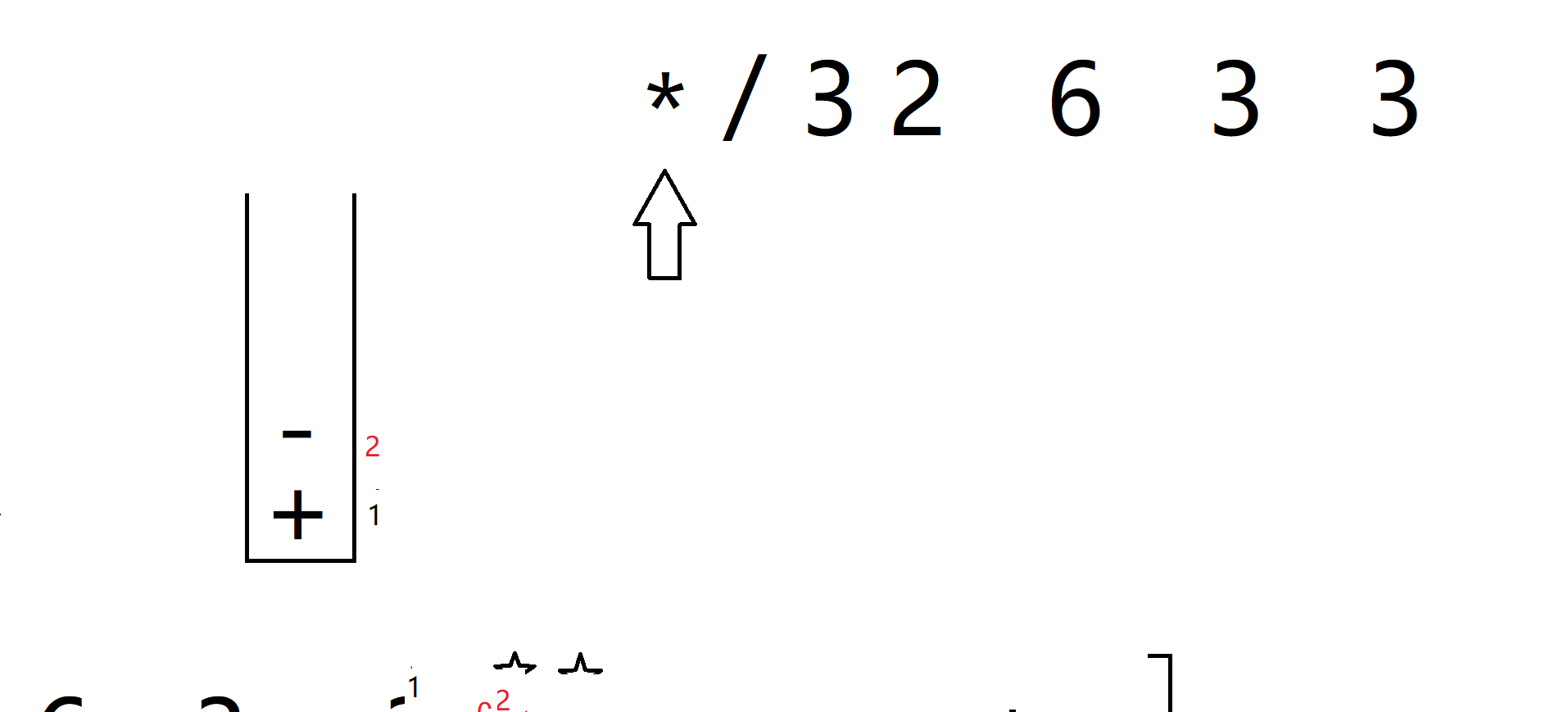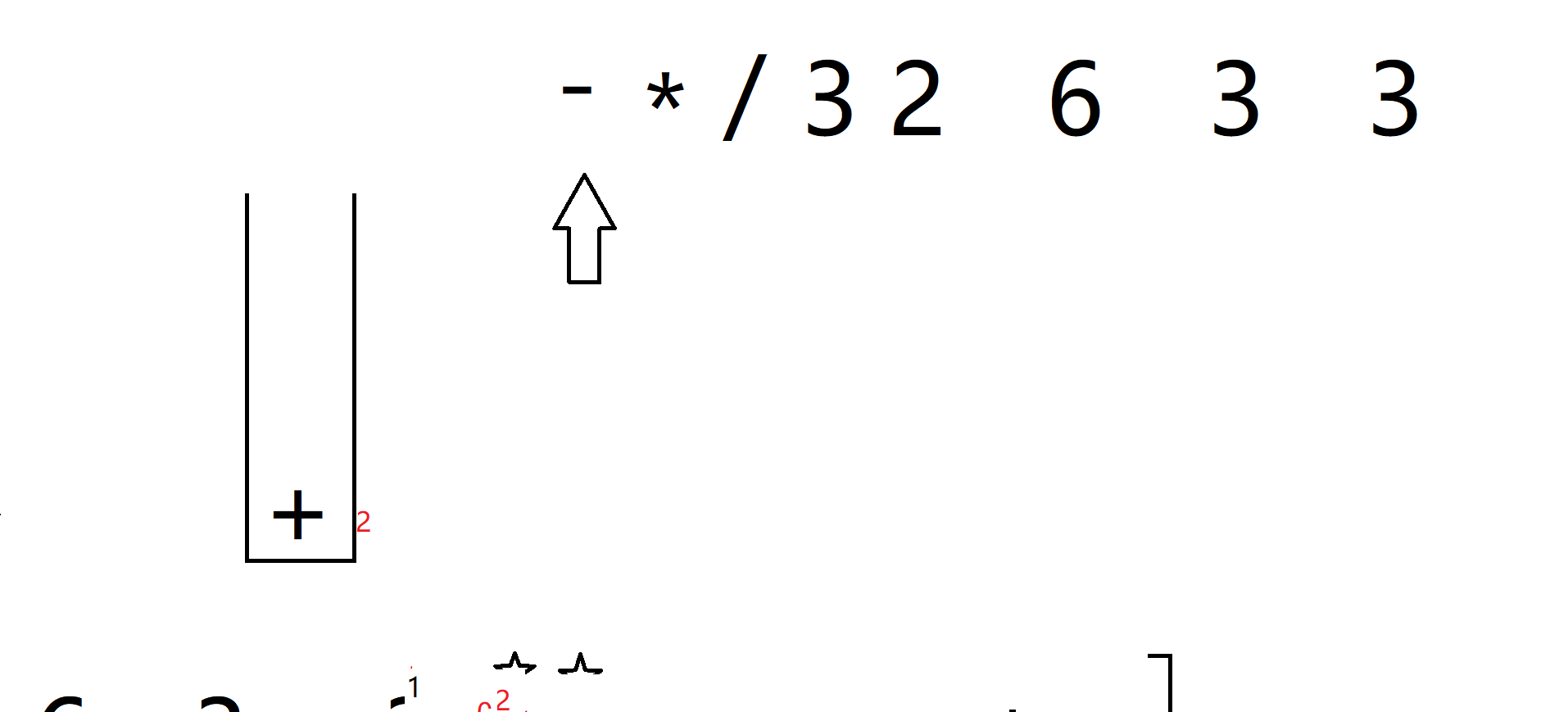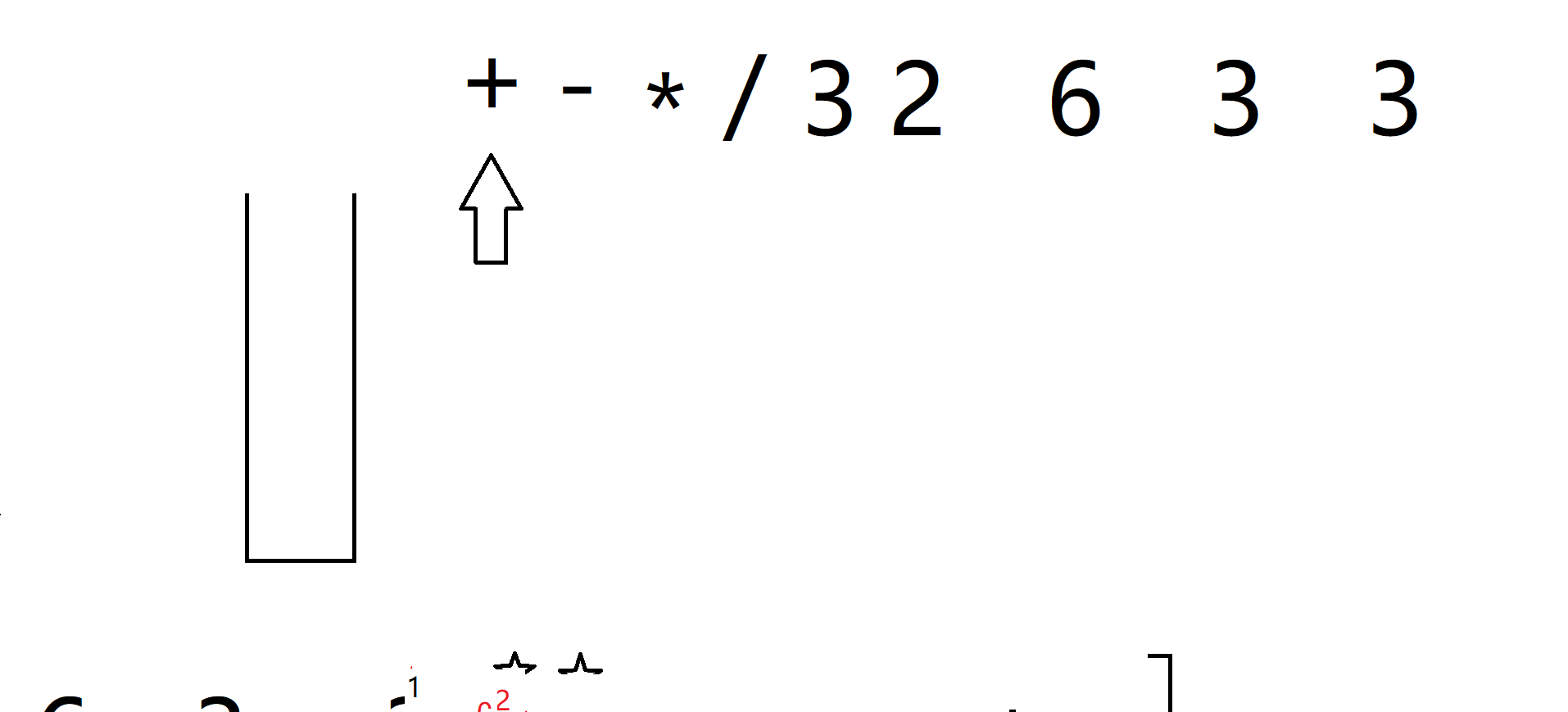对于表达式：32/6*3-3+
其前缀式为：+-*/32633
操作数的顺序不会改变，变的是运算符的顺序，并且在后缀式中，运算顺序从左往右，在前缀式中，正好反过来（但是运算符不一定正好反过来），这跟我们自己运算这两个表达式的顺序是一样的。转换的关键，就在如何找准操作符的两个操作数。
观察后缀表达式，对于32，越靠右边的操作数对32的运算迫切程度降低，总是要等到前面的运算符运算完才可以对32进行运算，由此想到栈结构来存储运算符。
总的思路如下：
指针从最后边扫描起，遇到操作符则存入栈中并且从表达式中删除该操作符，同时标记其匹配的操作数为0，如果遇到操作数，则应该对栈顶的操作符的配对操作数加一，当新来一个操作符时，对前面操作符的操作数配对期望降低，转而对新来的操作符先行配对操作数。当一个操作符成功配对两个操作数时，其配对期望程度为0，从栈中取出，插入到其两个操作数的前面，整体作为此时栈顶操作符新的操作数，并记录到栈顶操作符的操作数匹配数中。如果此时该栈顶操作符的操作数匹配恰好又为2，则再次取出，插入到其两个操作数前面，迭代此过程，直到栈顶操作符的操作数匹配数不足2或者栈空为止。

因为是如上的考虑，所以表达式存储采用链表，提高插入删除效率。
同时栈的data域要包含两个成员，其一为操作符，其二为该操作符匹配的操作数的数目。

下面是代码：

Status Convert(Expression *exp)
{//算法将后缀式exp转换为前缀式，如果后缀式不合法，则返回ERROR
SeqStack S;
InitStack(&S);

Revers(exp);//revers the expression
Ptr p = exp;
while (p->next!=NULL)//expression is still not over
{
if (Is_optr(p->next->ch))//is it an operator?
{
StackElem optr;
optr.optr = p->next->ch;
optr.its_oprd = 0;
Push(&S, optr);
Delet(exp, p);//delete the operator from expression
}
else
{
if (IsEmpty(&S))
return ERROR;//expression is illegal!
else
{
while (!IsEmpty(&S))
{
StackElem optr;
GetTop(&S, &optr);
Pop(&S);
if (++optr.its_oprd < 2)
{
Push(&S, optr);
break;
}
else
{
p = p->next;
Insert(exp, p, optr.optr);
}
}
p = p->next;
}
}
}
if (!IsEmpty(&S))
return ERROR;//expression is illegal!

Revers(exp);
return OK;
}
算法包含对后缀正确的判断，错误的后缀式（不算怪异字符，如不应该出现的‘】’‘&’等等）有两种情况，一种是取栈中元素时栈为空，说明此时后缀式存在非法的操作数过多的子表达式，第二种是表达式转换完栈不空，说明后缀式操作符过多。

如果你想运行上面的代码，你需要完整的源代码，如下：

#include "stdafx.h"
#include<stdio.h>
#include<iostream>
#include<malloc.h>
#define Status int
#define OVERFLOW -1
#define ERROR 0
#define OK 1
#define STACK_INI_SIZE 10//顺序栈初始化长度
#define STACK_INC_SIZE 2//顺序栈单次扩充长度

typedef struct StackElem
{
char optr;
int  its_oprd;//属于optr操作数出现的次数
}StackElem;//栈的元素类型
typedef struct SeqStack
{
StackElem  *base;
int        top;
int        capacity;//容量
}SeqStack;
typedef struct LinkList
{
char ch;//表达式元素
struct LinkList *next;
}Expression,ENode,*Ptr;

/*The major function*/
Status Convert(Expression *exp);
bool Is_optr(char ch);

/*Basic operation of SeqStack*/
//初始化栈
Status InitStack(SeqStack *S);
//获取栈顶元素的数据
Status GetTop(SeqStack *S, StackElem *optr);
//入栈
Status Push(SeqStack *S, StackElem optr);
//出栈
Status Pop(SeqStack *S);
//栈判空
bool IsEmpty(SeqStack *S);
//扩充栈空间
Status Extern(SeqStack *S);

/*Basic operation of Expression*/
//初始化表达式
Status InitExp(Expression **exp);
//构建表达式
Status Push_back(Expression *exp, char ch);
//插入节点
Status Insert(Expression *exp,Ptr p,char ch);
//删除节点
Status Delet(Expression *exp, Ptr p);
//逆置表达式
Status Revers(Expression *exp);
//输出表达式
Status Show(Expression *exp);

int main()
{
using namespace std;

ENode *exp;
InitExp(&exp);//初始化空表达式

cout << "Input a postfix expression(press @ to end input),thanks\n";
char ch;
while (cin >> ch, ch != '@')
{
Push_back(exp, ch);
}//expression like this  36*32/-

if (Convert(exp))
Show(exp);
else
cout << "Your expression is illegal\n";

return 0;
}

Status Convert(Expression *exp)
{//算法将后缀式exp转换为前缀式，如果后缀式不合法，则返回ERROR
SeqStack S;
InitStack(&S);

Revers(exp);//revers the expression
Ptr p = exp;
while (p->next!=NULL)//expression is still not over
{
if (Is_optr(p->next->ch))//is it an operator?
{
StackElem optr;
optr.optr = p->next->ch;
optr.its_oprd = 0;
Push(&S, optr);
Delet(exp, p);//delete the operator from expression
}
else
{
if (IsEmpty(&S))
return ERROR;//expression is illegal!
else
{
while (!IsEmpty(&S))
{
StackElem optr;
GetTop(&S, &optr);
Pop(&S);
if (++optr.its_oprd < 2)
{
Push(&S, optr);
break;
}
else
{
p = p->next;
Insert(exp, p, optr.optr);
}
}
p = p->next;
}
}
}
if (!IsEmpty(&S))
return ERROR;//expression is illegal!

Revers(exp);
return OK;
}

bool Is_optr(char ch)
{//判断ch是否是运算符
return ch == '+' || ch == '-' || ch == '*' || ch == '/';
}

Status InitStack(SeqStack *S)
{//初始化栈
S->base = (StackElem*)malloc(sizeof(StackElem)*STACK_INI_SIZE);
if (!S->base)
return OVERFLOW;

S->capacity = STACK_INI_SIZE;
S->top = 0;
return OK;
}

Status GetTop(SeqStack *S, StackElem *optr)
{//获取栈顶元素数据
if (IsEmpty(S))
return ERROR;

optr->optr = S->base[S->top - 1].optr;
optr->its_oprd = S->base[S->top - 1].its_oprd;
return OK;
}

Status Push(SeqStack *S, StackElem optr)
{
if (S->top == S->capacity && !Extern(S))
return OVERFLOW;

S->base[S->top].its_oprd = optr.its_oprd;
S->base[S->top].optr = optr.optr;
S->top++;
return OK;
}

Status Pop(SeqStack *S)
{
if (IsEmpty(S))
return ERROR;

S->top--;
return OK;
}

bool IsEmpty(SeqStack *S)
{
return S->top == 0;
}

Status Extern(SeqStack *S)
{
StackElem *newbase;
newbase = (StackElem*)realloc(S->base, (S->capacity + STACK_INC_SIZE) * sizeof(StackElem));
if (!newbase)
return OVERFLOW;

S->base = newbase;
S->capacity += STACK_INC_SIZE;
return OK;
}

Status InitExp(Expression **exp)
{//初始化空表达式
*exp = (ENode*)malloc(sizeof(ENode));
if (!(*exp))
return OVERFLOW;

(*exp)->next = NULL;
return OK;
}

Status Push_back(Expression *exp, char ch)
{//尾部插入
ENode *p = exp, *s;

while (p->next != NULL)
{
p = p->next;
}
s = (ENode*)malloc(sizeof(ENode));
if (!s)
return OVERFLOW;

s->next = NULL;
s->ch = ch;
s->next = p->next;
p->next = s;
return OK;
}

Status Insert(Expression *exp, Ptr p, char ch)
{//在指针p的后面插入节点ch
ENode *s = (ENode*)malloc(sizeof(ENode));
if (!s)
return OVERFLOW;

s->next = NULL;
s->ch = ch;
s->next = p->next;
p->next = s;
return OK;
}

Status Delet(Expression *exp, Ptr p)
{//删除P后面的节点
if (p->next == NULL)
return ERROR;

ENode *q = p->next;
p->next = q->next;
free(q);
return OK;
}

Status Revers(Expression *exp)
{//逆置表达式exp
if (exp->next == NULL || exp->next->next == NULL)
return OK;

ENode *p, *s;
p = exp->next->next;
exp->next->next = NULL;
s = p;
while (p != NULL)
{
s = s->next;
p->next = exp->next;
exp->next = p;
p = s;
}
return OK;
}

Status Show(Expression *exp)
{//输出表达式

ENode *p = exp;
while (p->next != NULL)
{
std::cout << p->next->ch << ' ';
p = p->next;
}
return OK;
}

不足之处请提出来，希望能帮到你！
展开全文• 我们用存储后缀表达式中的数据部分，当遇到操作符时就取出中的栈顶两个元素，检测操作符的类型，并进行相应的计算（这里要注意的是，对于除法运算，栈顶的两个元素的位置得区分）。 如下所示： 三、...中缀表达式 枚举 结构体
• #include /* 栈实现后缀表达式的计算,因为是后缀表达式计算所以只要定义一个OPND操作数栈，遇到运算符直接取前两个操作数运算后并压入栈即可*/#include #include #define overflow 1typedef struct Stack{ ...数据结构 c
• 运用后缀表达式进行计算的具体做法： 建立一个S 。从左到右读表达式，如果读到操作数就将它压入栈S中，如果读到n元运算符(即需要参数个数为n的运算符)则取出由栈顶向下的n项按操作数运算，再将运算的结果代替原...
• 不想过多解释了，其实就是将普通的表达式画成树的形式...#include //后缀表达式实现的应用） #include "stack.h" int main() { s X; init(&X); char s,*tp,tc; scanf("%s",s); tp = s; //
• 题目： 使用栈实现后缀表达式计算 要求： 使用栈实现后缀表达式计算，其中，在后缀表达式中，输入的数字为整数，且为正数，数字、符号之间用逗号隔开，整个后缀表达式用“#”表示结束。 输入样例： 11 2 3 + *# 输出...算法 数据结构 c++ stack
• 今天在学数据结构，自己撸一段用实现后缀表达式求值的代码，能改进的地方还有很多，在此先mark一下package StackPractice;import java.util.Scanner; import java.util.Stack; import java.util.regex.Pattern;...java 数据结构
• #include<iostream> using namespace std; template <class T> class Stack{ public: void clear(); bool push(); bool pop(T& item); bool ttop(T& item); bool isEmpty();...tem.数据结构
• 1，中缀表达式转后缀表达式 1）遇到操作数直接拼到字符串 2）遇到操作符 a,遇到左括号 b,遇到右括号 c,遇到加减 d,遇到乘除 2，计算后缀表达式 #中缀表达式转后缀表达式 def middle2after(s=""): rule = {...
• 主要介绍了PHP实现基于栈的后缀表达式求值功能,简单描述了后缀表达式的概念并结合实例形式分析了php使用栈实现后缀表达式求值的相关操作技巧,需要的朋友可以参考下
• 主要为大家详细介绍了C++利用栈实现中缀表达式转后缀表达式，文中示例代码介绍的非常详细，具有一定的参考价值，感兴趣的小伙伴们可以参考一下
• 书籍在线网址http://interactivepython.org/runestone/static/pythonds/index.html 中文翻译书籍：... 将中缀表达式转换为后缀表达式 ...　中缀表达式（上图，一般书写）转换为后缀表达式（A...
• 栈实现中缀表达式到后缀表达式的转换 InfixToSuffix 用C语言编写 code blocks开发
• C语言版中缀表达式转后缀表达式 I 通常，用户输入的表达是为中缀表达式，通过算法，将中缀表达式转化为后缀表达式，然后利用存储操作数，遇到operator，弹出中的操作数进行相应的运算后再圧，直至表达式运算...
• 后缀表达式表示为：8 4 + 6 2 * - 例题2 中缀表达式：（70 + 30） *20 + 10 / 2 - 3 后缀表达式： 70 30 + 20 * 10 2 / + 3 - 例题3 中缀表达式： 10 * 3 +（3-1）* 2 - 10 / 2 后缀表达式：10 3 * 3 1 - 2 * + 10 2...
• 利用栈实现中缀表达式转换成后缀表达式 #include "stdio.h" #include "stdlib.h" #include "math.h" #define OK 1 #define ERROR 0 #define STACK_INIT_DATA 20 #define STACKINCREMENT 10 typedef char ...
• 后缀表达式中缀表达式转后缀表达式后缀表达式的计算 中缀表达式转后缀表达式 后缀表达式： 所有的符号都是在要计算的数字后面，并且没有括号。 规则： 从左到右遍历中缀表达式每个数字和符号，若是数字就输出，若是...
•c语言
• 用自定义的栈实现算术表达式求值 基本思想就是把中缀表达式通过栈转换为后缀表达式，然后利用后缀表达式求值。当然可以换成STL里的栈。但如果都用STL了还学什么数据结构嘛。看不懂留言。 #include <iostream> ...算法 字符串 数据结构
• 形参设计：传进2个字符数组的地址，第一个为中缀表达式的数组地址，将此 中缀表达式转化为后缀表达式赋给第二个地址所在的字符数组。   while(*exp!=’\0’) { switch(*exp) { (1)扫描为‘(’:进栈，exp++,...数据结构与算法
• 先了解一下什么是中缀和后缀表达式中缀表达式就是我们平常写的算式，比如2*（3+4）-8/2，它对应的后缀表达式是234+*82/-。不要着急，接下来我们看看是怎么得到后缀表达式的。当遇到数字时，直接输出，遇到符号入栈，......